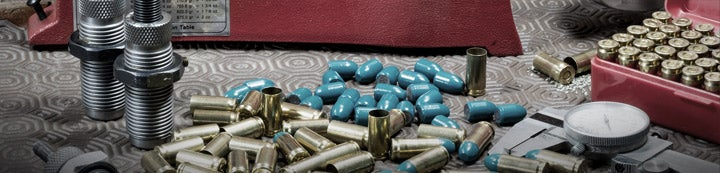1 - 1 of 1 Posts

#### Ciocc

·
##### Registered
Joined
·
1 Posts
Discussion Starter · ·
R = KPS x RF / Wr + C

Where:

KPS = ( Wb x V. ) ^2 / 4013

RF = ( V. / Wb ) x 75 + 1.75 = Recoil Factor

Wb = weight of bullet
V. = velocity, with the comma replaced with a decimal point
Wr = weight of rifle
C =
-2 for Wb < 100 gr
-1.5 for Standard cartridges, and for Short Magnums Wb < 250 gr
0 for Magnums Wb < 200 gr, and for Short Magnums Wb = 250 gr
2 for Magnums Wb > 180 gr

Example:
Standard Wb x V. xx Wr xx KPS x RF Wr xxC xxx R
.30-06 - 150 - 2.910 - 8 --- 47.5 x 3.2 / 8 - 1.5 = 17.5 ft lbs

R on Chuck Hawks' Table of Recoils = 17.6 ft lbs

This is simple math: times,square,divide, then divide,times,add, then times,divide, add/subtract. If you have a three- memory calculator, you can automate the calculations by having M1=4013, M2=75, M3=1.75.

Online recoil calculators require the weight of the powder charge: this formula doesn't. All you need is the bullet weight and muzzle velocity of your cartridge, and the weight of your rifle. The results should be within 3 ft lbs of the averaged recoil number in a recoil table ( except for very large magnums, like the 111 ft lbs of a .460 Weatherby!)

One can obtain the same velocity for a bullet from two different powders, which have different weights, and so produce different recoils. This difference can be at least as much as 3 ft lbs. Different cartridge manufacturers will use different powders, and their powders and charges may be changed over time. Recoil tables are by necessity tables of averaged values, but your current factory load may well differ by a few ft lbs. So don't get attached to any absolute value from a table. Unless you open a cartridge and weigh the powder, this formula is as good as a recoil table.

Chuck Hawks developed a Killing Power Score (KPS) formula for any cartridge, equal to the Energy at impact times the SD of the bullet times the Area of the caliber. This score has many uses, including determining the suitability of a load for what maximum game size at a given range, and the maximum range for a given game size. I came up with a simplified expression for the KPS formula.

I've wondered whether the recoil of a cartridge was proportional to its killing power. When I looked at the saami recoil formula, I realized that it was almost identical to the KPS formula, except that the recoil formula included two more variables, the powder weight and the rifle weight. I plugged my very compact KPS formula into the saami recoil formula, and came up with this new recoil formula for factory cartridges, whose powder weight is unknown.

1) For implications and applications of Chuck Hawks' KPS formula, check out his vast, multi-interest site.

2) A way to test the utility of this recoil formula is to pick a cartridge, a bullet weight, and a muzzle velocity. Go to the Hodgdon powder site. From among the powders of the three powder manufacturers, pick the smallest and largest loads that give about the right velocity for that bullet. Take these two weights to an online recoil calculator, and plug them in. Then try this formula, and compare the results.

You can also keep a recoil table honest in this way. Sometimes errors happen, like the 7 lb rifle that should have been 8 lb. Another time, one cartridge with two names (9.3x66/.370 Sako magnum) had two entries differing by 3 ft lbs, adjusting for a common rifle weight.

In general, most results with this formula should agree with a table within a ft lb, with the rest within two ft lbs. I hope that this recoil formula is of some use to the shooting community. --Craig Mooslin

1 - 1 of 1 Posts